# RD Sharma Solutions for Class 7 Maths Chapter 24 Data Handling - III (Constructions of Bar Graphs)

The pdf of RD Sharma Solutions for Class 7 Chapter 24 Data Handling – III (Constructions of Bar Graphs) are provided here. The subject experts at BYJU’S outline the concepts in a clear and precise manner based on the IQ level of students. The solutions to all questions in RD Sharma solutions are given here in a detailed and step by step way to help the students understand more effectively.

By solving this chapter, the students will understand how to draw the bar graph, a double bar graph for the given data. RD Sharma Solutions for Class 7 are given here which include the answers to all the questions present in this chapter. Let us have a look at some of the concepts that are being discussed in this chapter.

• Definition and meaning of bar graphs
• Steps to construct bar graphs

## Download the PDF of RD Sharma Solutions For Class 7 Maths Chapter 24 Data Handling – III (Constructions of Bar graphs)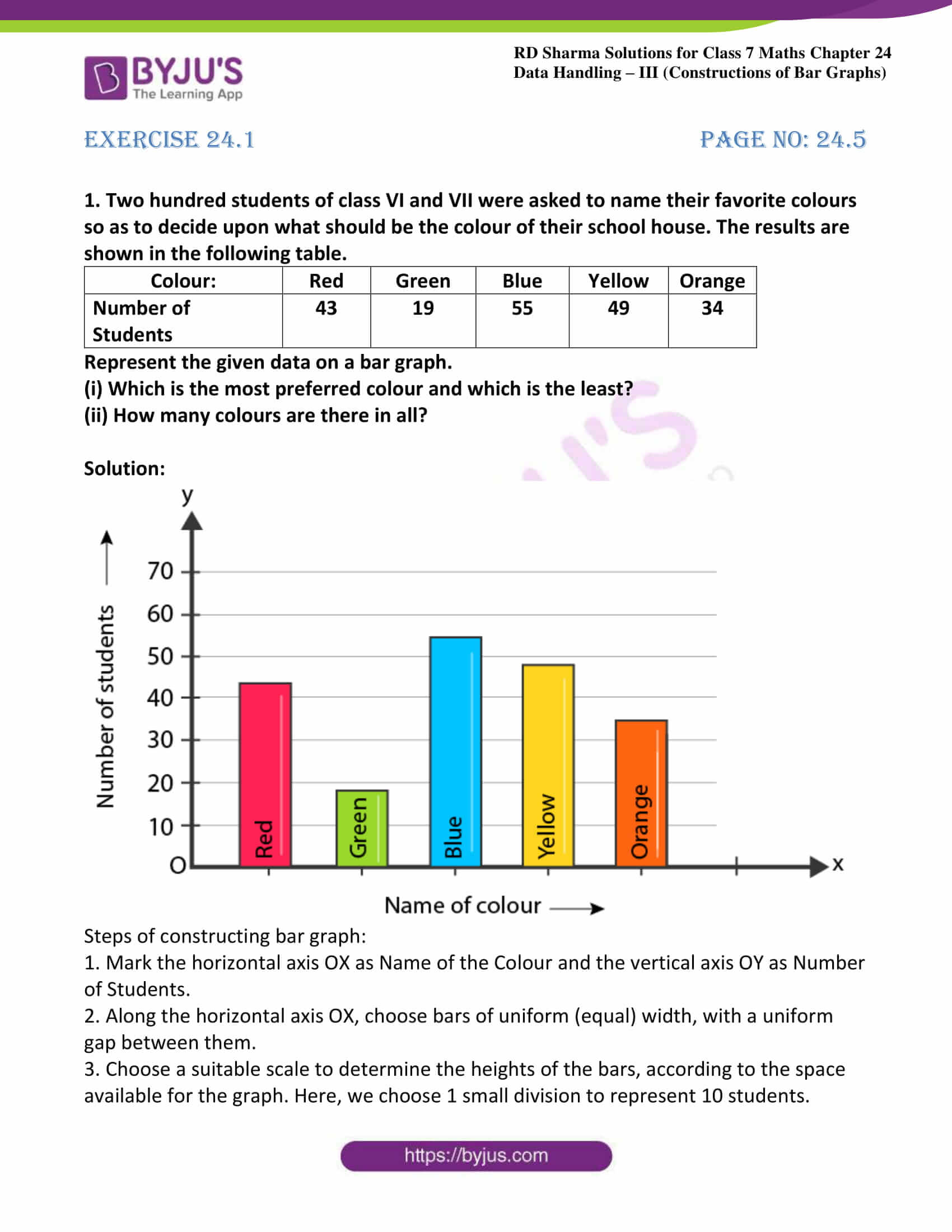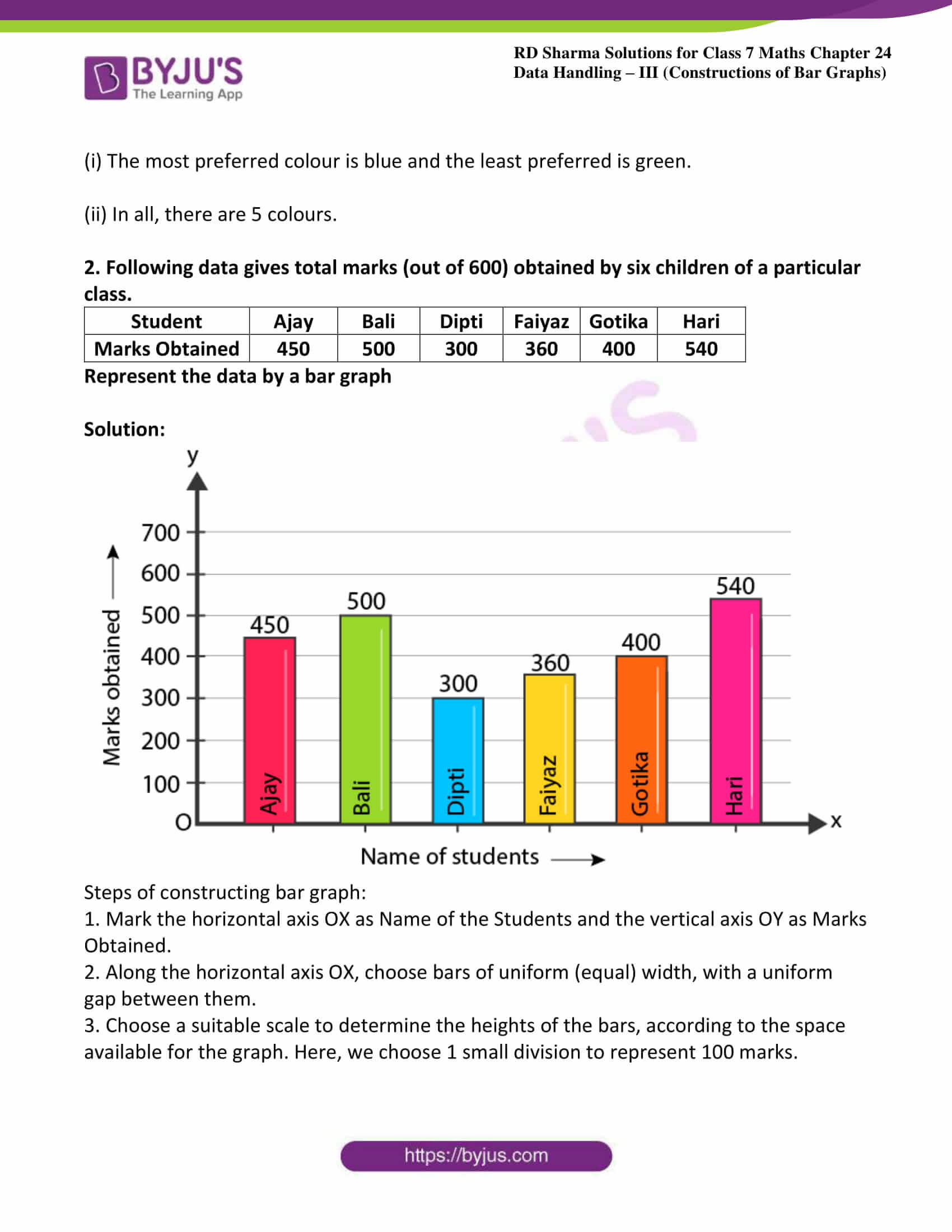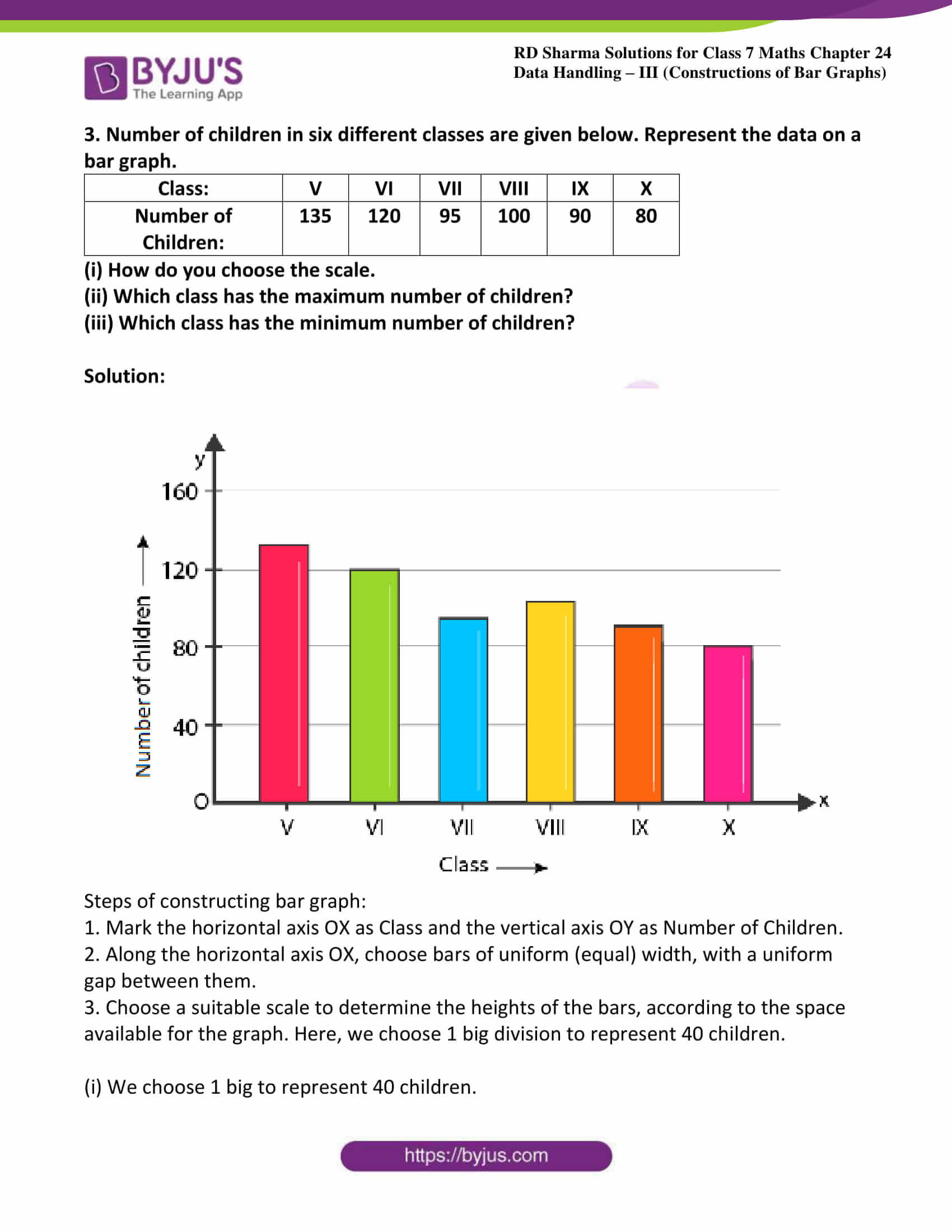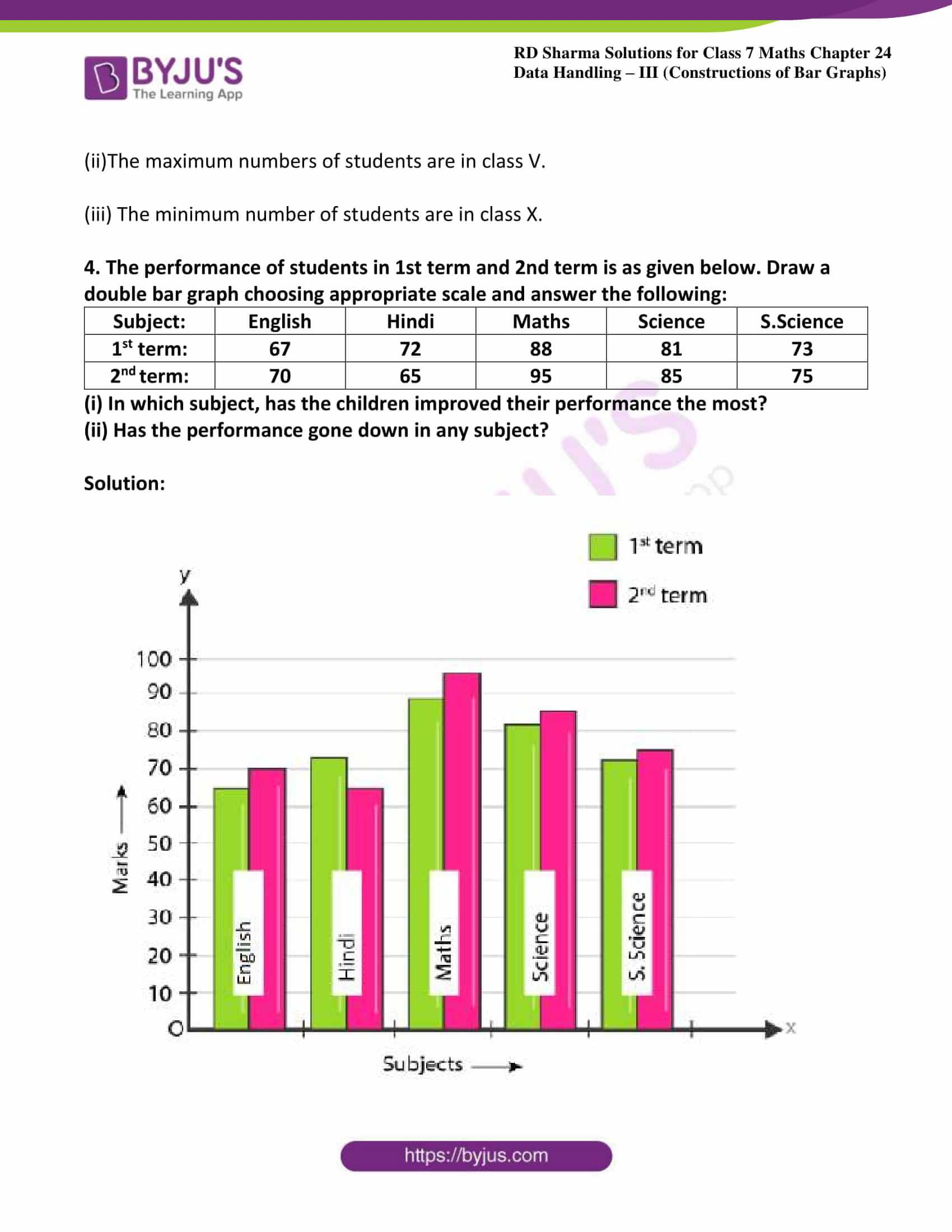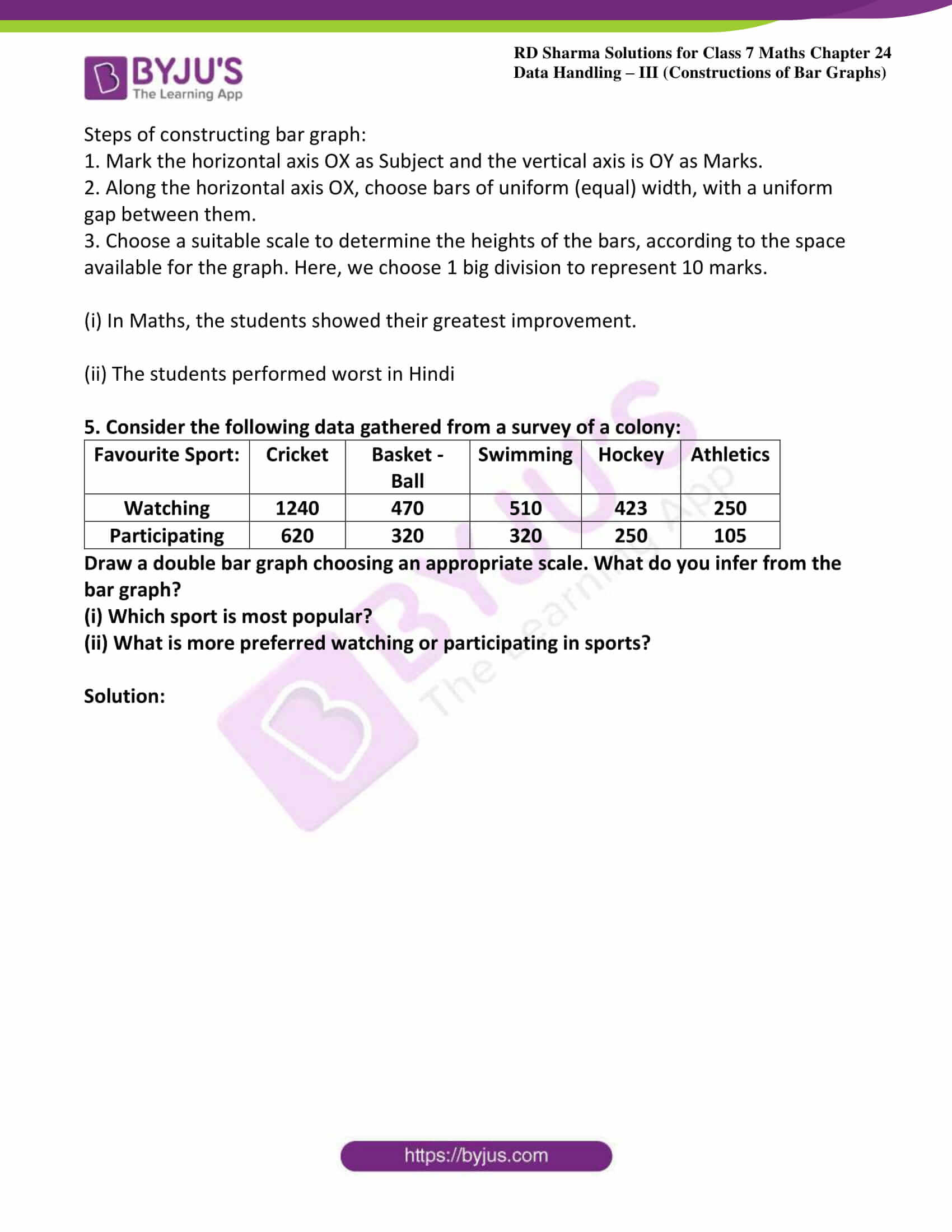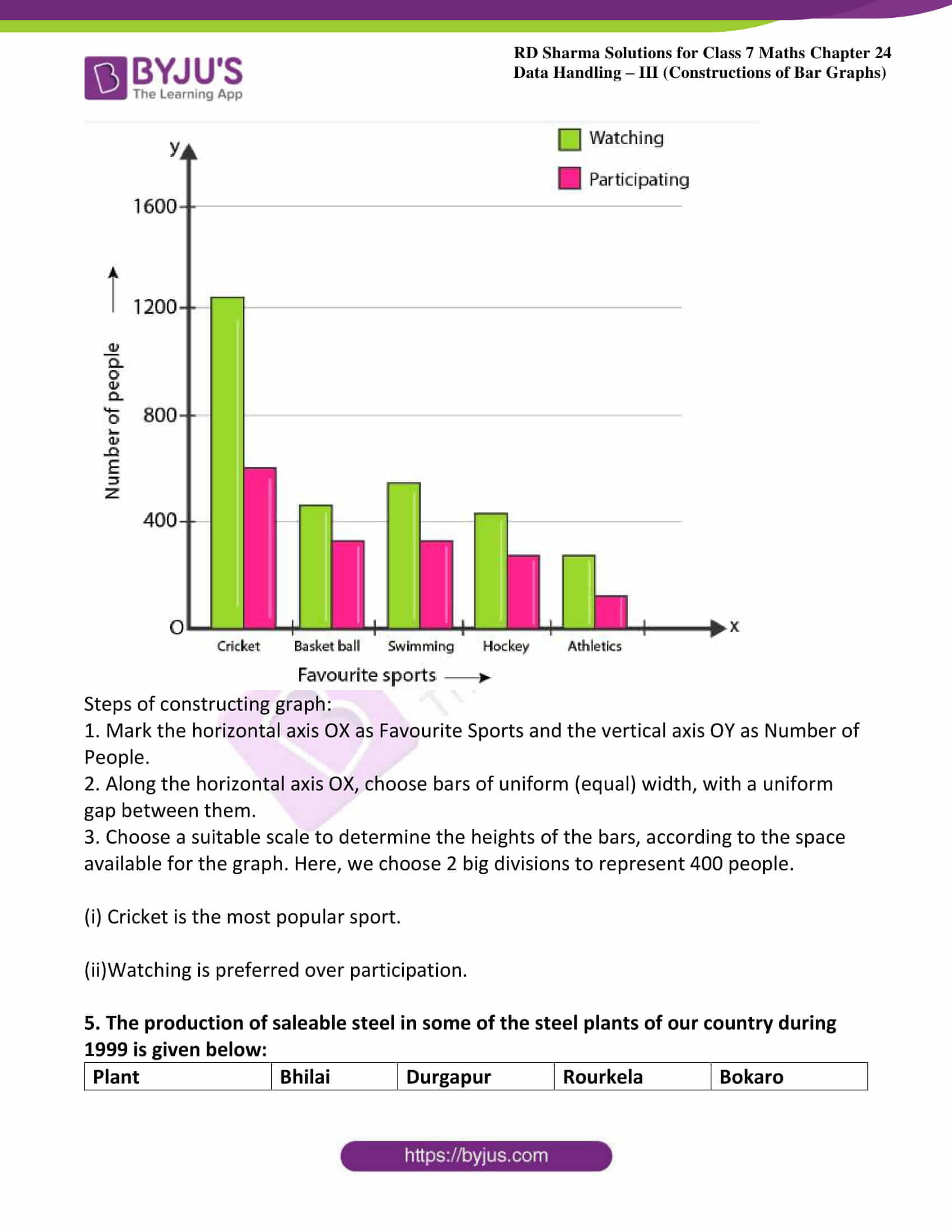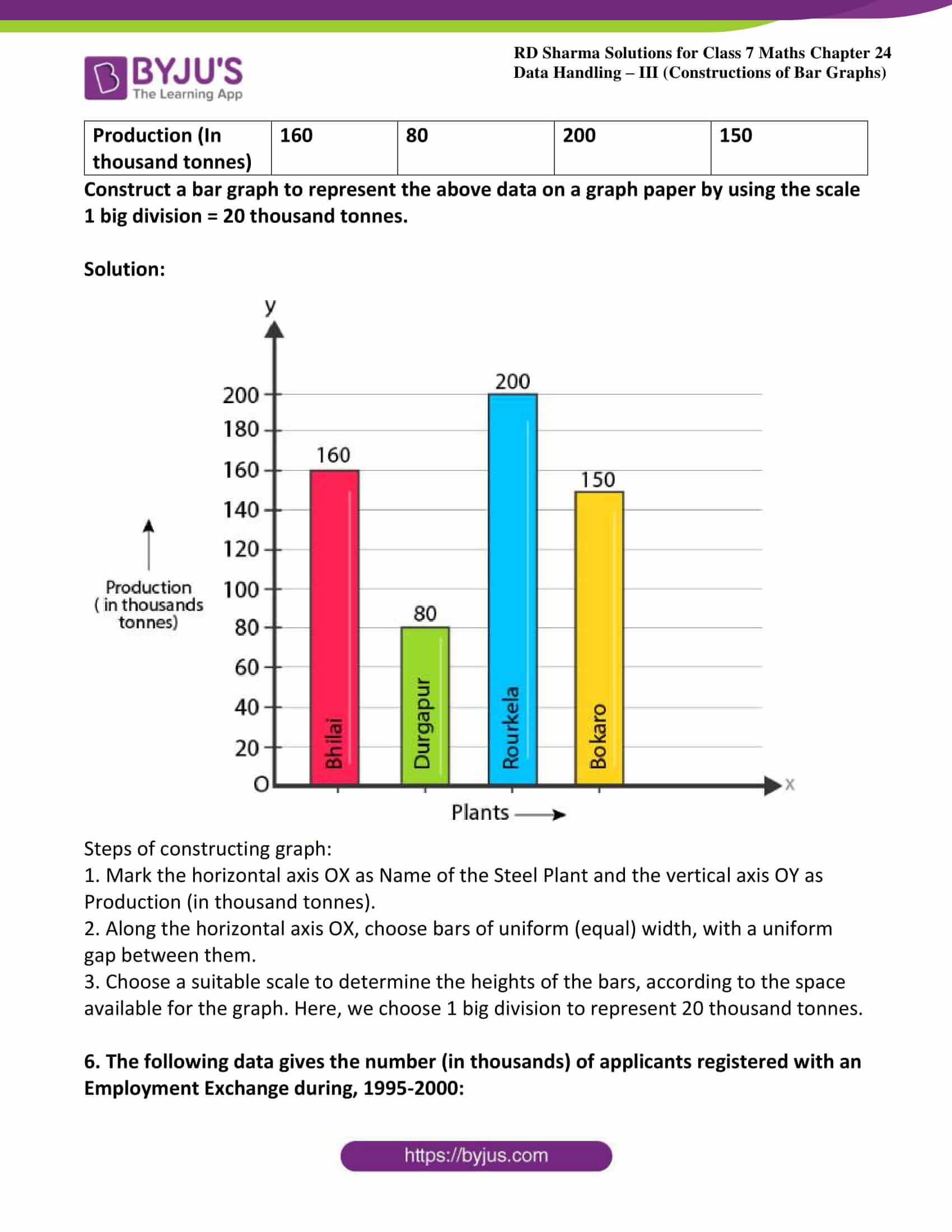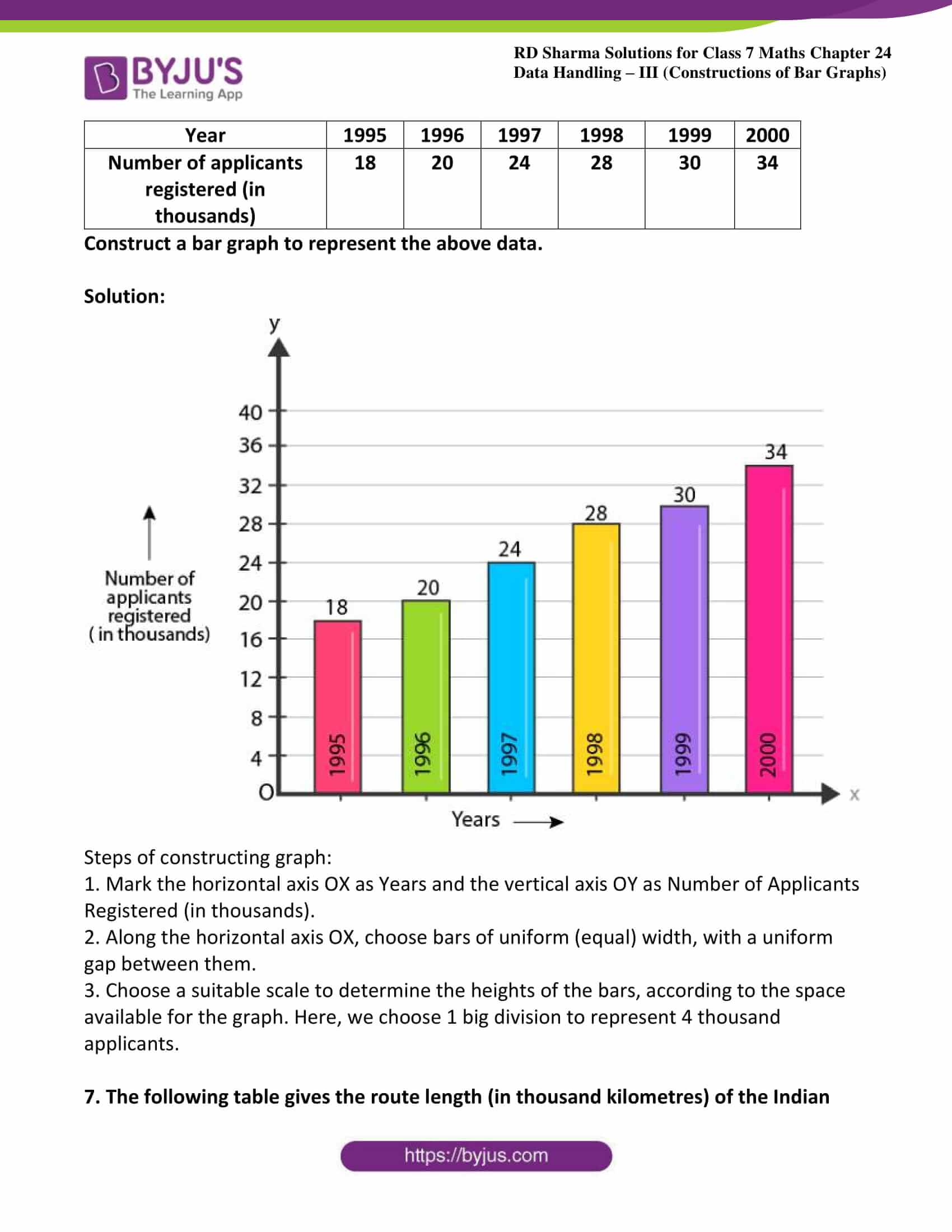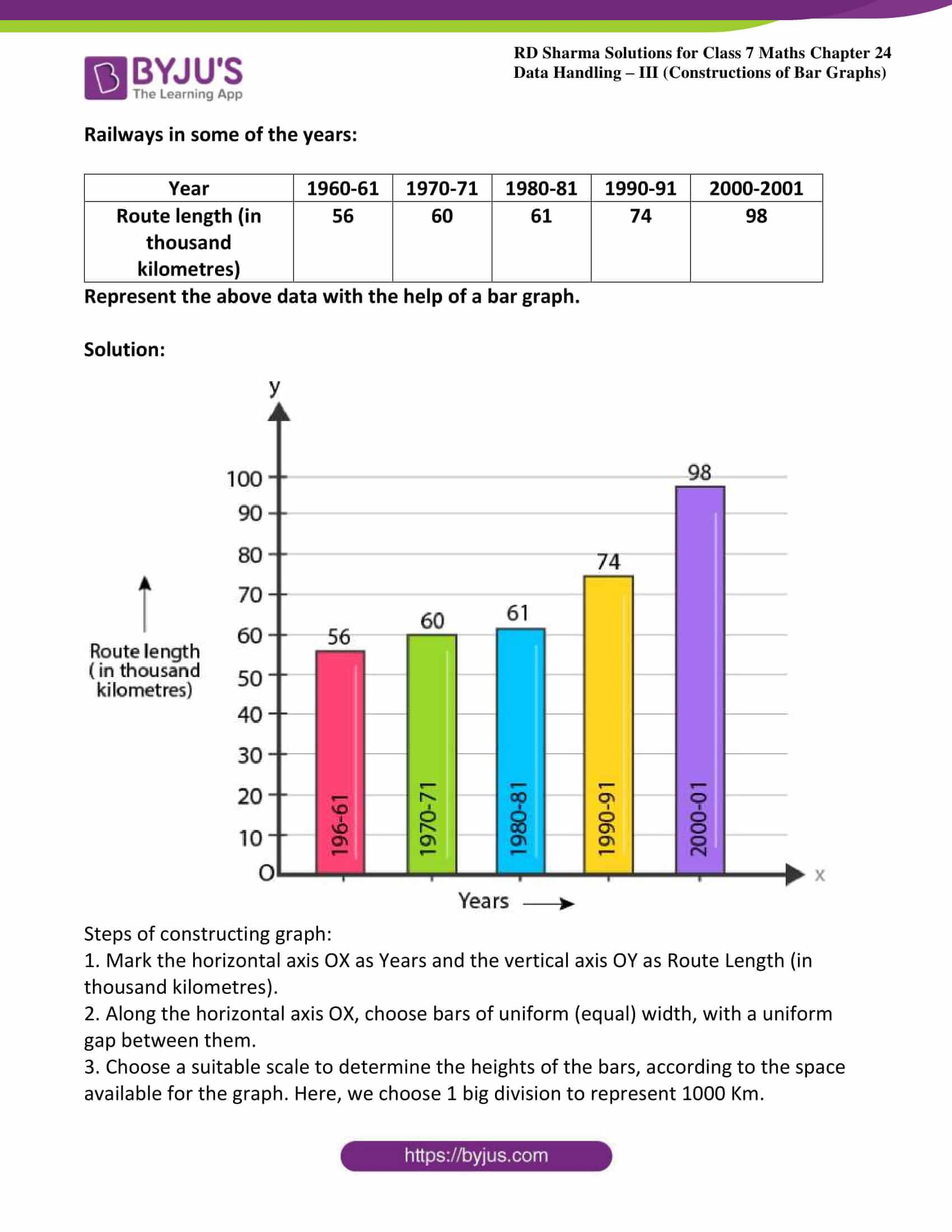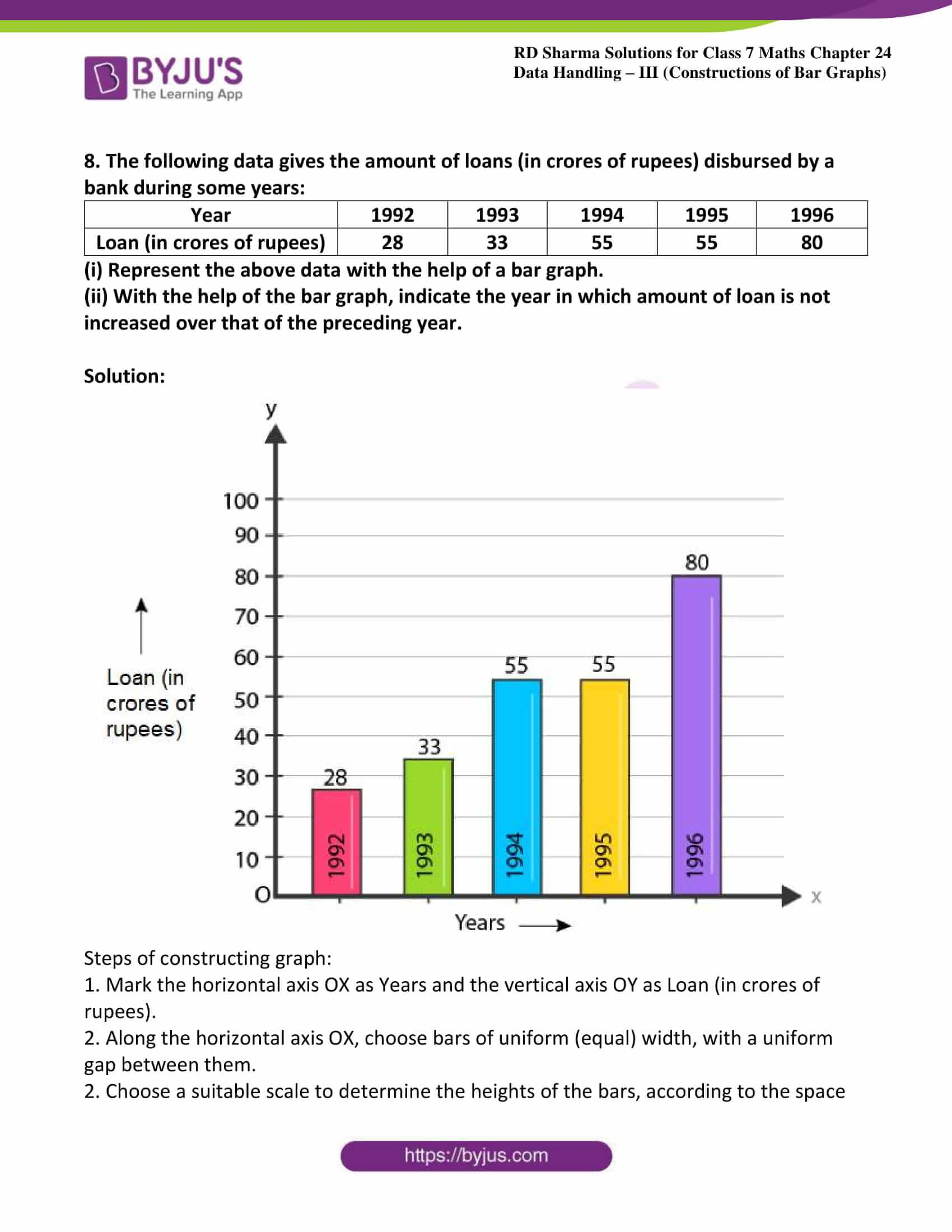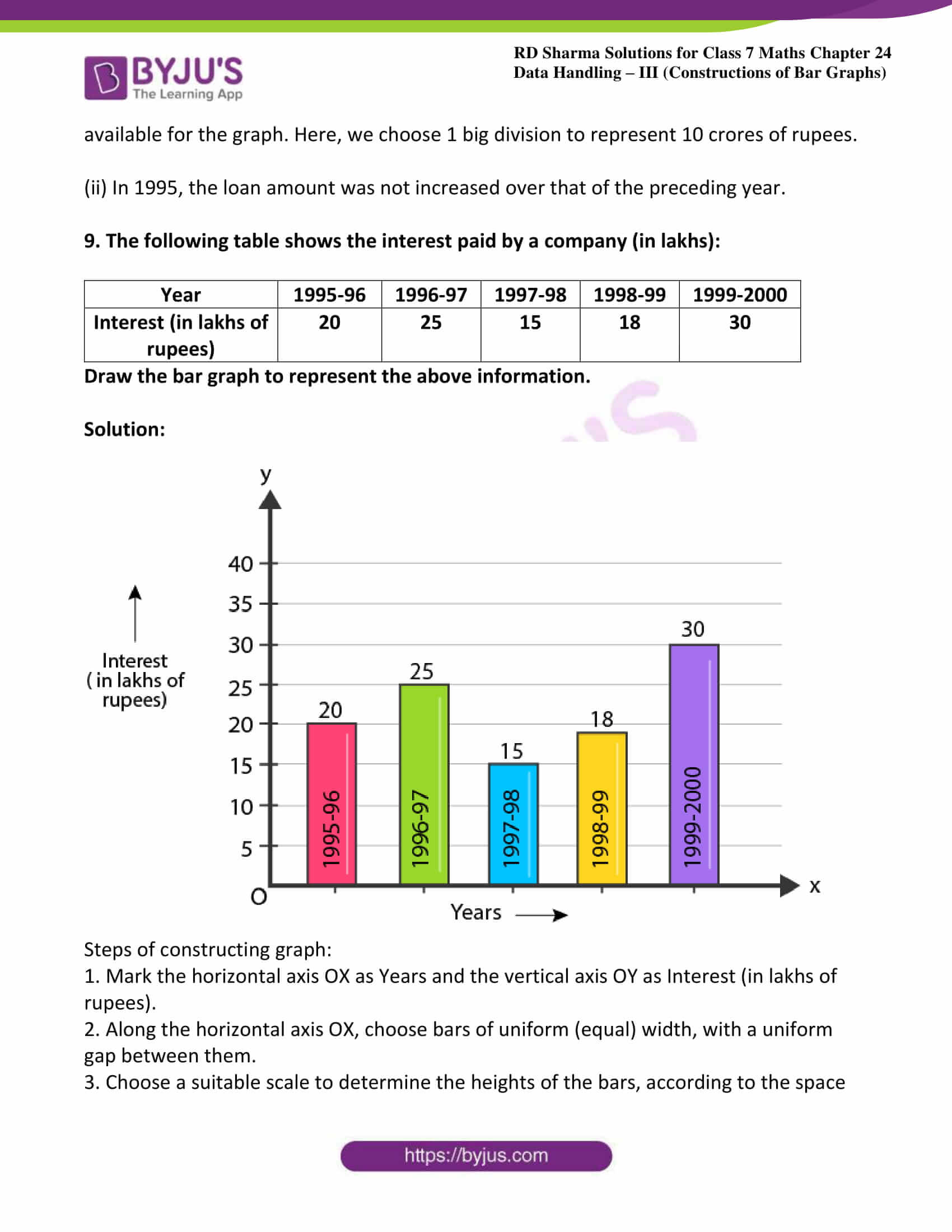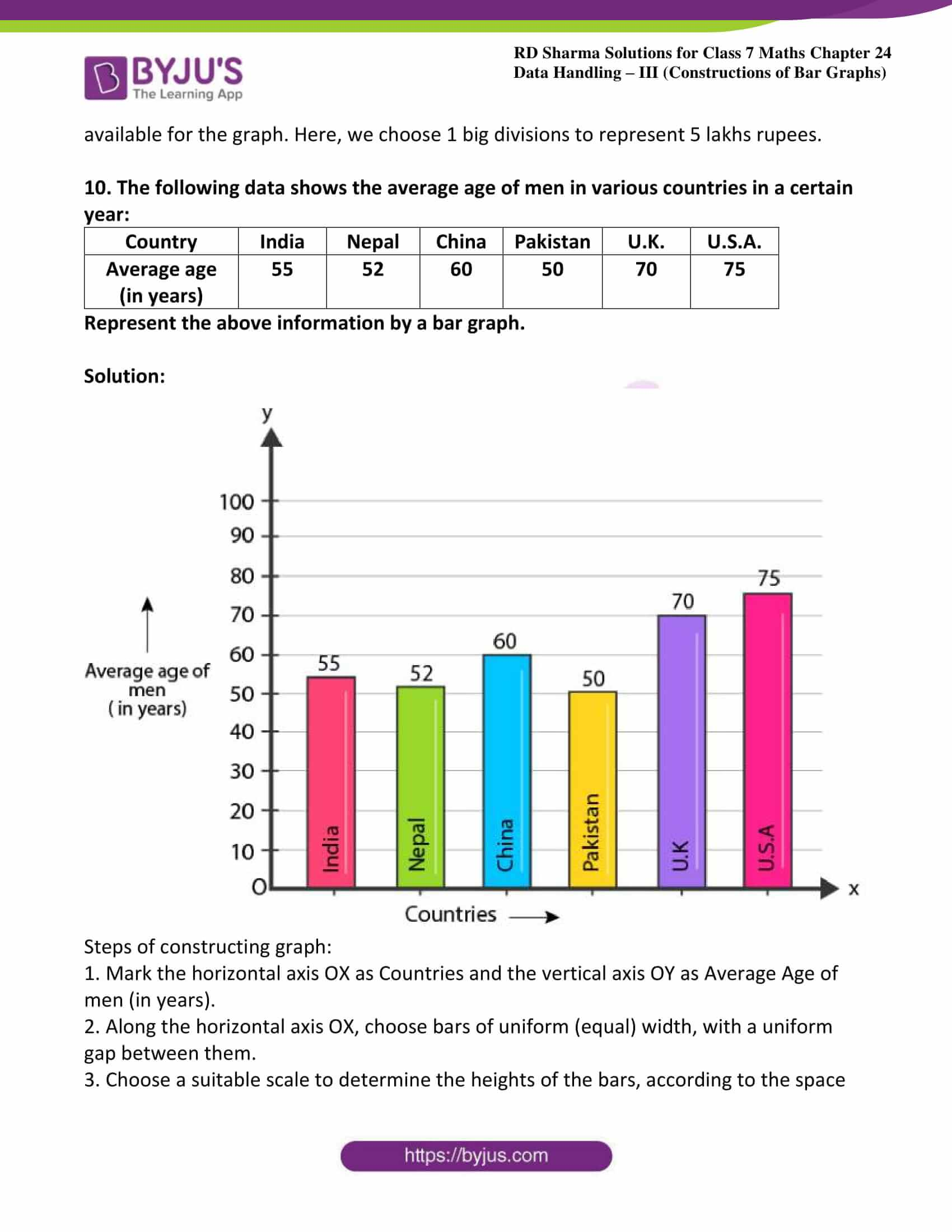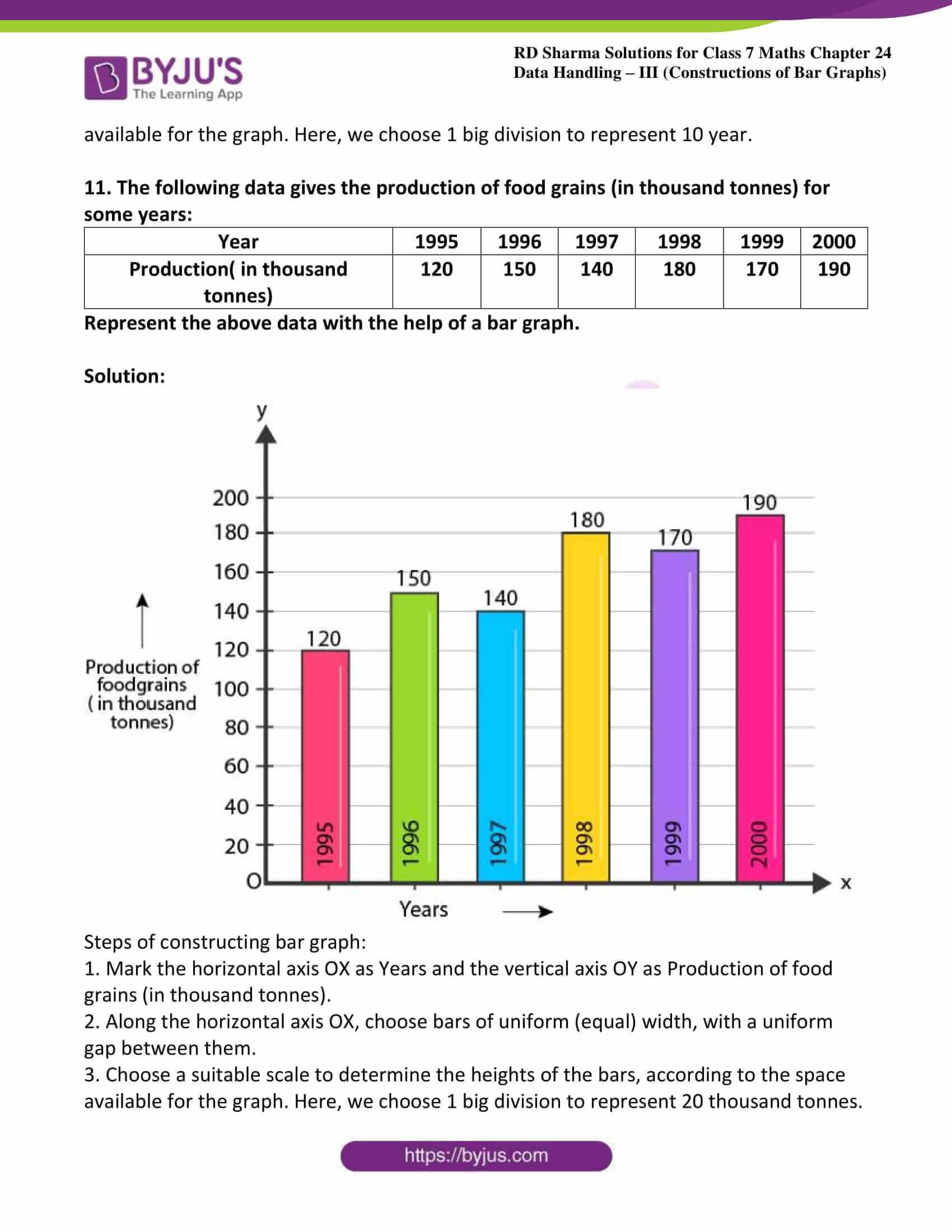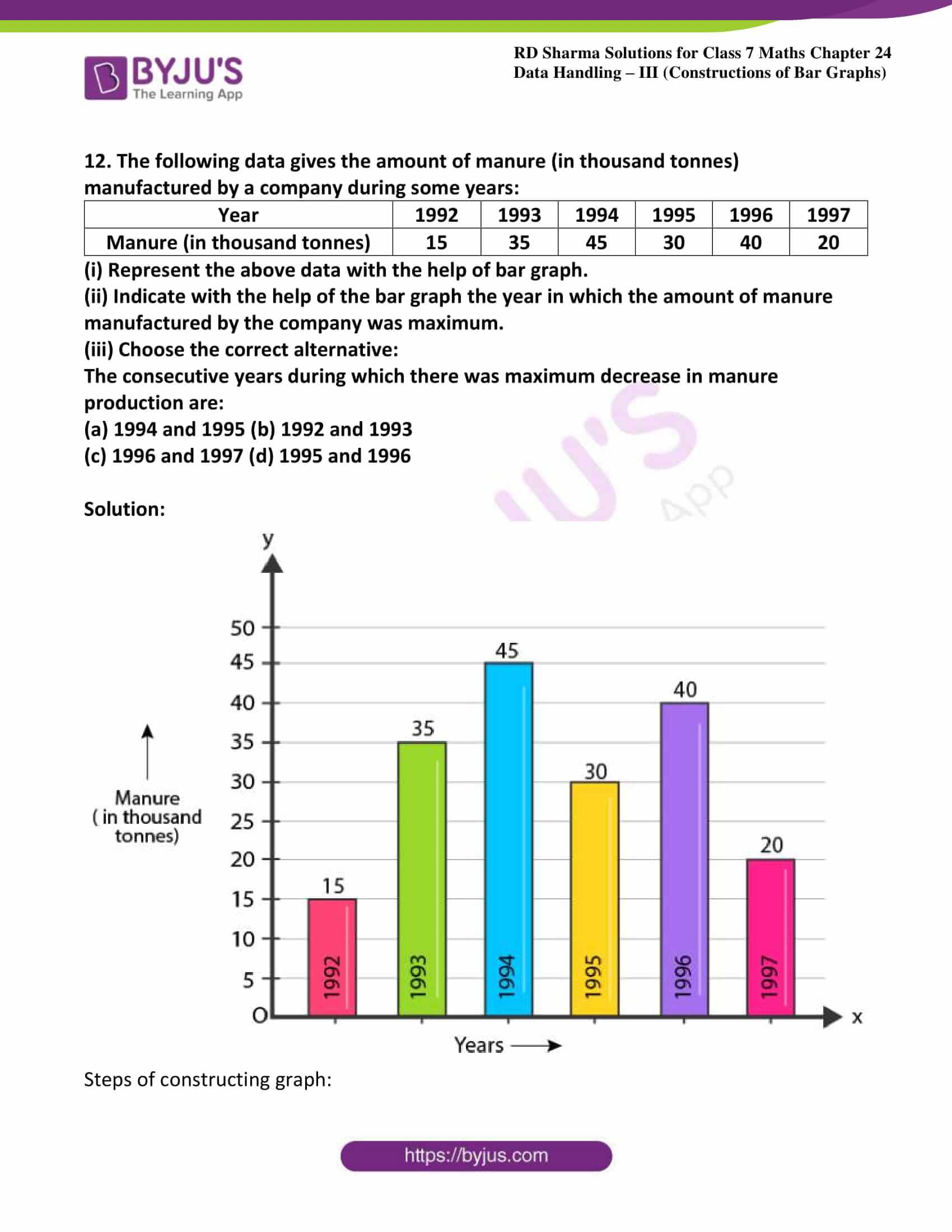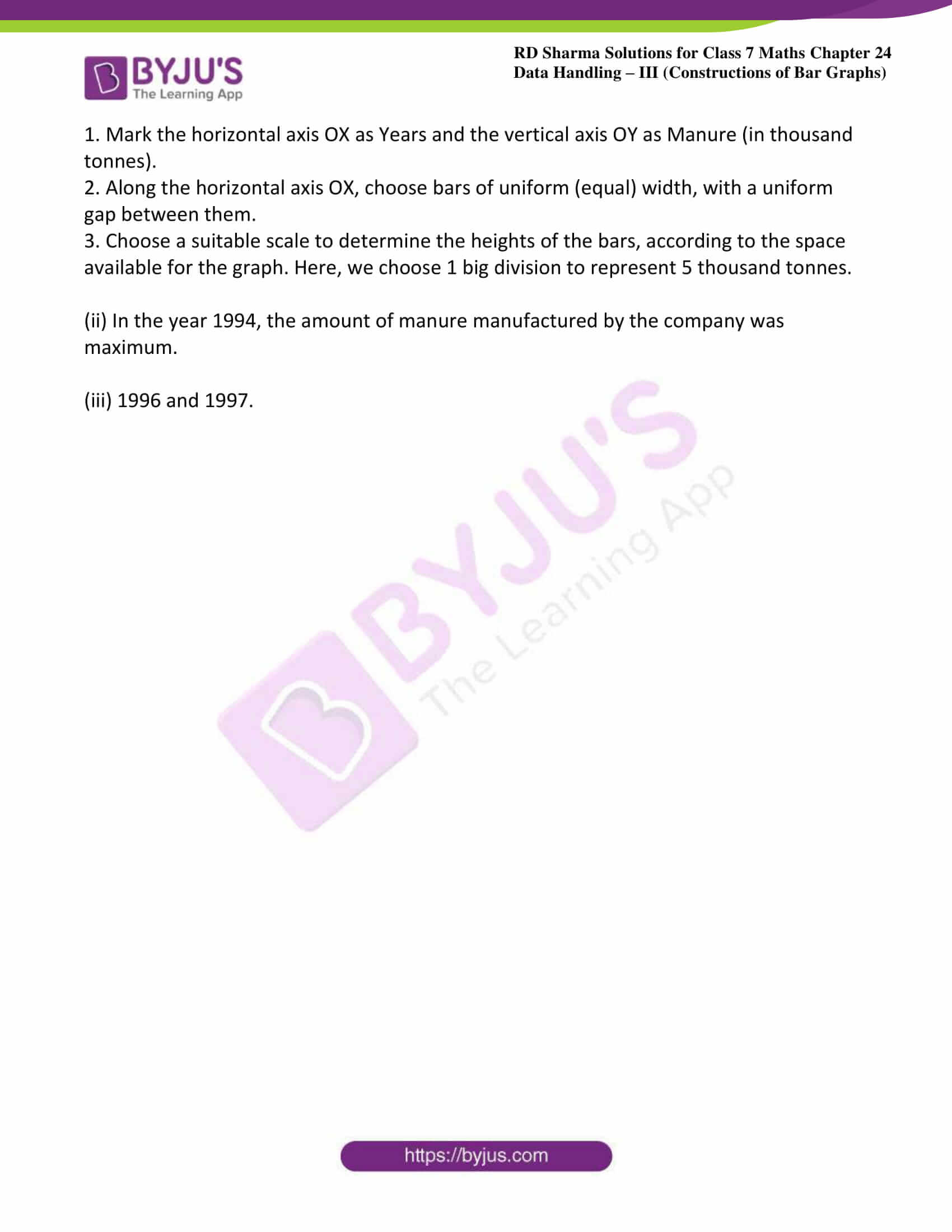### Access answers to Maths RD Sharma Solutions For Class 7 Chapter 24 – Data Handling – III (Constructions of Bar graphs)

1. Two hundred students of class VI and VII were asked to name their favorite colours so as to decide upon what should be the colour of their school house. The results are shown in the following table.

 Colour: Red Green Blue Yellow Orange Number of Students 43 19 55 49 34

Represent the given data on a bar graph.

(i) Which is the most preferred colour and which is the least?

(ii) How many colours are there in all?

Solution: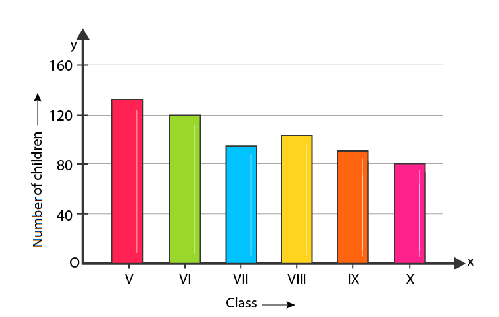Steps of constructing bar graph:

1. Mark the horizontal axis OX as Name of the Colour and the vertical axis OY as Number of Students.

2. Along the horizontal axis OX, choose bars of uniform (equal) width, with a uniform gap between them.

3. Choose a suitable scale to determine the heights of the bars, according to the space available for the graph. Here, we choose 1 small division to represent 10 students.

(i) The most preferred colour is blue and the least preferred is green.

(ii) In all, there are 5 colours.

2. Following data gives total marks (out of 600) obtained by six children of a particular class.

 Student Ajay Bali Dipti Faiyaz Gotika Hari Marks Obtained 450 500 300 360 400 540

Represent the data by a bar graph

Solution: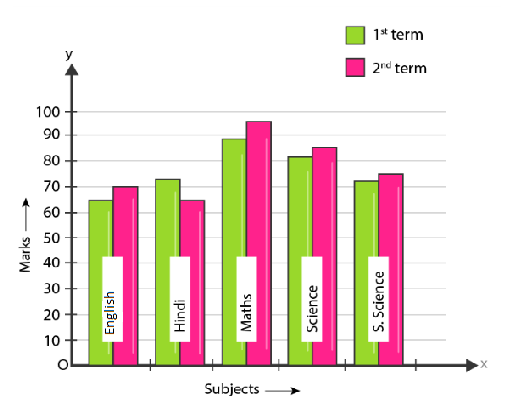Steps of constructing bar graph:

1. Mark the horizontal axis OX as Name of the Students and the vertical axis OY as Marks Obtained.

2. Along the horizontal axis OX, choose bars of uniform (equal) width, with a uniform gap between them.

3. Choose a suitable scale to determine the heights of the bars, according to the space available for the graph. Here, we choose 1 small division to represent 100 marks.

3. Number of children in six different classes are given below. Represent the data on a bar graph.

 Class: V VI VII VIII IX X Number of Children: 135 120 95 100 90 80

(i) How do you choose the scale.

(ii) Which class has the maximum number of children?

(iii) Which class has the minimum number of children?

Solution:Steps of constructing bar graph:

1. Mark the horizontal axis OX as Class and the vertical axis OY as Number of Children.

2. Along the horizontal axis OX, choose bars of uniform (equal) width, with a uniform gap between them.

3. Choose a suitable scale to determine the heights of the bars, according to the space available for the graph. Here, we choose 1 big division to represent 40 children.

(i) We choose 1 big to represent 40 children.

(ii)The maximum numbers of students are in class V.

(iii) The minimum number of students are in class X.

4. The performance of students in 1st term and 2nd term is as given below. Draw a double bar graph choosing appropriate scale and answer the following:

 Subject: English Hindi Maths Science S.Science 1st term: 67 72 88 81 73 2nd term: 70 65 95 85 75

(i) In which subject, has the children improved their performance the most?

(ii) Has the performance gone down in any subject?

Solution:Steps of constructing bar graph:

1. Mark the horizontal axis OX as Subject and the vertical axis is OY as Marks.

2. Along the horizontal axis OX, choose bars of uniform (equal) width, with a uniform gap between them.

3. Choose a suitable scale to determine the heights of the bars, according to the space available for the graph. Here, we choose 1 big division to represent 10 marks.

(i) In Maths, the students showed their greatest improvement.

(ii) The students performed worst in Hindi

5. Consider the following data gathered from a survey of a colony:

 Favourite Sport: Cricket Basket – Ball Swimming Hockey Athletics Watching 1240 470 510 423 250 Participating 620 320 320 250 105

Draw a double bar graph choosing an appropriate scale. What do you infer from the bar graph?

(i) Which sport is most popular?

(ii) What is more preferred watching or participating in sports?

Solution: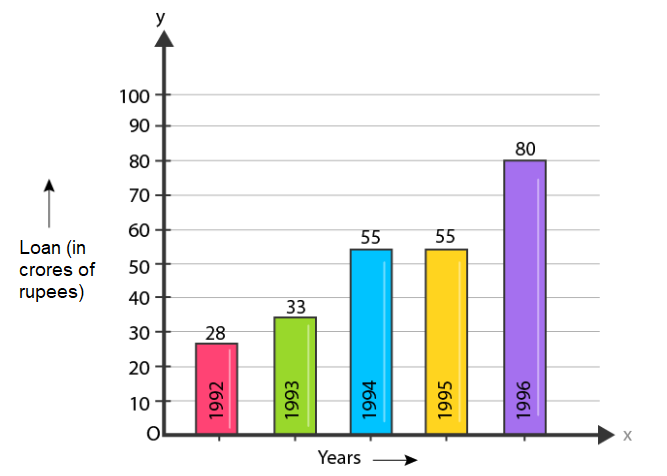Steps of constructing graph:

1. Mark the horizontal axis OX as Favourite Sports and the vertical axis OY as Number of People.

2. Along the horizontal axis OX, choose bars of uniform (equal) width, with a uniform gap between them.

3. Choose a suitable scale to determine the heights of the bars, according to the space available for the graph. Here, we choose 2 big divisions to represent 400 people.

(i) Cricket is the most popular sport.

(ii)Watching is preferred over participation.

5. The production of saleable steel in some of the steel plants of our country during 1999 is given below:

 Plant Bhilai Durgapur Rourkela Bokaro Production (In thousand tonnes) 160 80 200 150

Construct a bar graph to represent the above data on a graph paper by using the scale 1 big division = 20 thousand tonnes.

Solution: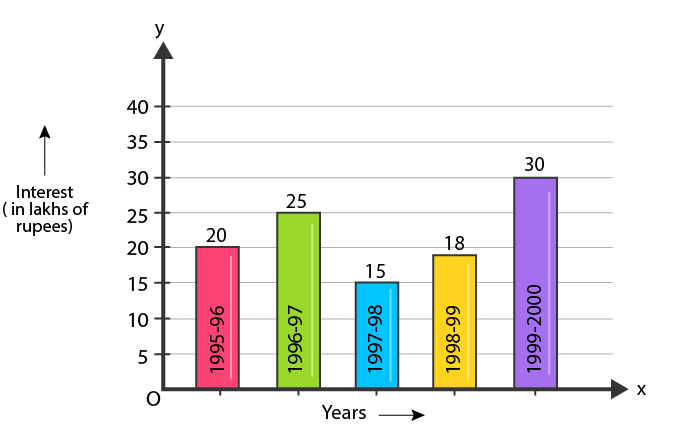Steps of constructing graph:

1. Mark the horizontal axis OX as Name of the Steel Plant and the vertical axis OY as Production (in thousand tonnes).

2. Along the horizontal axis OX, choose bars of uniform (equal) width, with a uniform gap between them.

3. Choose a suitable scale to determine the heights of the bars, according to the space available for the graph. Here, we choose 1 big division to represent 20 thousand tonnes.

6. The following data gives the number (in thousands) of applicants registered with an Employment Exchange during, 1995-2000:

 Year 1995 1996 1997 1998 1999 2000 Number of applicants registered (in thousands) 18 20 24 28 30 34

Construct a bar graph to represent the above data.

Solution: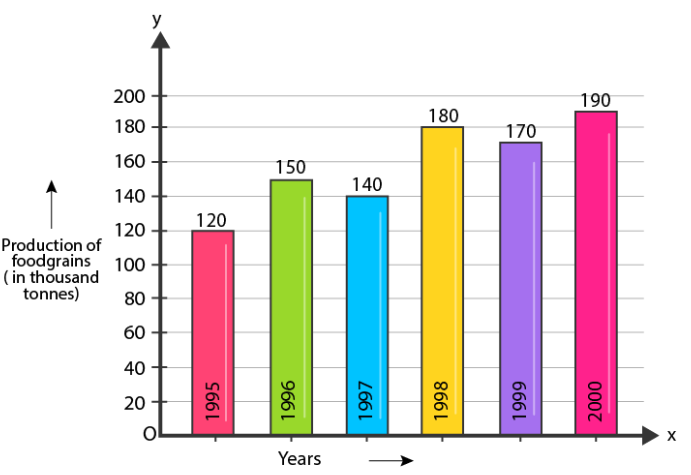Steps of constructing graph:

1. Mark the horizontal axis OX as Years and the vertical axis OY as Number of Applicants Registered (in thousands).

2. Along the horizontal axis OX, choose bars of uniform (equal) width, with a uniform gap between them.

3. Choose a suitable scale to determine the heights of the bars, according to the space available for the graph. Here, we choose 1 big division to represent 4 thousand applicants.

7. The following table gives the route length (in thousand kilometres) of the Indian Railways in some of the years:

 Year 1960-61 1970-71 1980-81 1990-91 2000-2001 Route length (in thousand kilometres) 56 60 61 74 98

Represent the above data with the help of a bar graph.

Solution: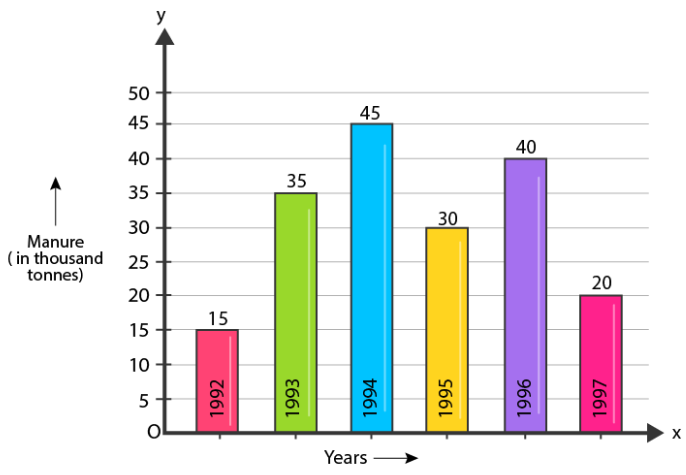Steps of constructing graph:

1. Mark the horizontal axis OX as Years and the vertical axis OY as Route Length (in thousand kilometres).

2. Along the horizontal axis OX, choose bars of uniform (equal) width, with a uniform gap between them.

3. Choose a suitable scale to determine the heights of the bars, according to the space available for the graph. Here, we choose 1 big division to represent 1000 Km.

8. The following data gives the amount of loans (in crores of rupees) disbursed by a bank during some years:

 Year 1992 1993 1994 1995 1996 Loan (in crores of rupees) 28 33 55 55 80

(i) Represent the above data with the help of a bar graph.

(ii) With the help of the bar graph, indicate the year in which amount of loan is not increased over that of the preceding year.

Solution:Steps of constructing graph:

1. Mark the horizontal axis OX as Years and the vertical axis OY as Loan (in crores of rupees).

2. Along the horizontal axis OX, choose bars of uniform (equal) width, with a uniform gap between them.

2. Choose a suitable scale to determine the heights of the bars, according to the space available for the graph. Here, we choose 1 big division to represent 10 crores of rupees.

(ii) In 1995, the loan amount was not increased over that of the preceding year.

9. The following table shows the interest paid by a company (in lakhs):

 Year 1995-96 1996-97 1997-98 1998-99 1999-2000 Interest (in lakhs of rupees) 20 25 15 18 30

Draw the bar graph to represent the above information.

Solution:Steps of constructing graph:

1. Mark the horizontal axis OX as Years and the vertical axis OY as Interest (in lakhs of rupees).

2. Along the horizontal axis OX, choose bars of uniform (equal) width, with a uniform gap between them.

3. Choose a suitable scale to determine the heights of the bars, according to the space available for the graph. Here, we choose 1 big divisions to represent 5 lakhs rupees.

10. The following data shows the average age of men in various countries in a certain year:

 Country India Nepal China Pakistan U.K. U.S.A. Average age (in years) 55 52 60 50 70 75

Represent the above information by a bar graph.

Solution: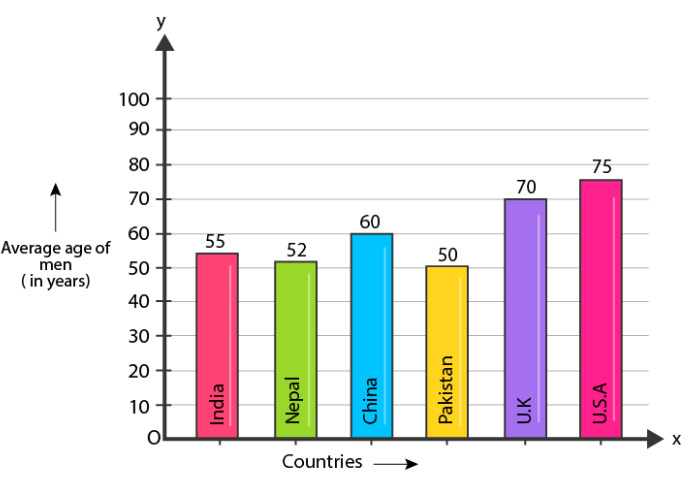Steps of constructing graph:

1. Mark the horizontal axis OX as Countries and the vertical axis OY as Average Age of men (in years).

2. Along the horizontal axis OX, choose bars of uniform (equal) width, with a uniform gap between them.

3. Choose a suitable scale to determine the heights of the bars, according to the space available for the graph. Here, we choose 1 big division to represent 10 year.

11. The following data gives the production of food grains (in thousand tonnes) for some years:

 Year 1995 1996 1997 1998 1999 2000 Production( in thousand tonnes) 120 150 140 180 170 190

Represent the above data with the help of a bar graph.

Solution:Steps of constructing bar graph:

1. Mark the horizontal axis OX as Years and the vertical axis OY as Production of food grains (in thousand tonnes).

2. Along the horizontal axis OX, choose bars of uniform (equal) width, with a uniform gap between them.

3. Choose a suitable scale to determine the heights of the bars, according to the space available for the graph. Here, we choose 1 big division to represent 20 thousand tonnes.

12. The following data gives the amount of manure (in thousand tonnes) manufactured by a company during some years:

 Year 1992 1993 1994 1995 1996 1997 Manure (in thousand tonnes) 15 35 45 30 40 20

(i) Represent the above data with the help of bar graph.

(ii) Indicate with the help of the bar graph the year in which the amount of manure manufactured by the company was maximum.

(iii) Choose the correct alternative:

The consecutive years during which there was maximum decrease in manure production are:

(a) 1994 and 1995 (b) 1992 and 1993

(c) 1996 and 1997 (d) 1995 and 1996

Solution:Steps of constructing graph:

1. Mark the horizontal axis OX as Years and the vertical axis OY as Manure (in thousand tonnes).

2. Along the horizontal axis OX, choose bars of uniform (equal) width, with a uniform gap between them.

3. Choose a suitable scale to determine the heights of the bars, according to the space available for the graph. Here, we choose 1 big division to represent 5 thousand tonnes.

(ii) In the year 1994, the amount of manure manufactured by the company was maximum.

(iii) 1996 and 1997.

1. Krish

Nice